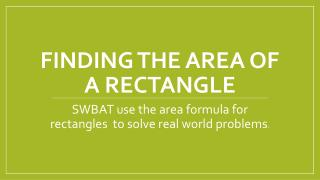DownloadDownload PresentationFinding the Area of a Rectangle

# Finding the Area of a Rectangle

Télécharger la présentation## Finding the Area of a Rectangle

- - - - - - - - - - - - - - - - - - - - - - - - - - - E N D - - - - - - - - - - - - - - - - - - - - - - - - - - -
##### Presentation Transcript

1. Finding the Area of a Rectangle SWBAT use the area formula for rectangles to solve real world problems.

2. Do Now • Linda has ¾ gallon of ice cream. She put an equal amount of ice cream into 6 bowls. How much ice cream was in each bowl? • 1/8 gallon B. ¼ gallon C. 4/9 gallon D. 9/2 gallon 2. One ticket to the high school football game is \$5.50. A season ticket for one person to attend all six home games is \$30.00. How much money would a family of four save if they bought four season tickets? • \$3.00 B. \$12.00 C. \$12.50 D. \$30.00 3. A trout is 22 inches long and a flounder is 20 inches long. How much longer is the trout? 4. Mrs Murphy ate 2/5 of a pizza and Ms Dalton ate 1/8 of a pizza. How much of the pizza is left?

3. What is area? Take 1 minute to write: What is area? What does the word mean? When have you seen it before? Define in your own words. Area: The size of a surface. The amount of space inside the boundary of a flat object such as a rectangle or a triangle.

4. How are area and perimeter different? Area is the space inside a 2 dimensional shape. Perimeter is the distance around the shape. Example on board

5. Area of a Rectangle • To find the area of a rectangle, we multiply the length times the width. In the rectangle shown, we have dimensions of 5 x 8. Area means the amount of squares it takes to cover the flat surface. In this case, it takes 40. (We also know that 5 x 8 = 40) This rectangle has an area of 40 units2

6. Find the area Find the area of the following rectangles. 12 units 24 sq units 2 units 27 sq units 3 units 9 units 5 units 25 sq units 5 units

7. Turn and talk • With the person sitting next to you, come up with 3 real world examples of when we could need to calculate area. Be prepared to share. You have 5 minutes.

8. Independent Practice

9. Exit Ticket • What is the area of a rectangle with dimensions of 7.5 and 4? • Explain how to find area. • Which of the following is NOT an area problem. • Paul is painting a wall with dimensions of 9 x 12. How much paint will he need? • Tina’s yard is 25 feet by 30 feet. How much fencing will she need to fence in her yard? • Randy is getting new carpeting. If his room is 13 feet wide and 24.5 feet long, how much carpeting will he need?

10. Homework • Create and solve a word problem for finding the area of a rectangle.